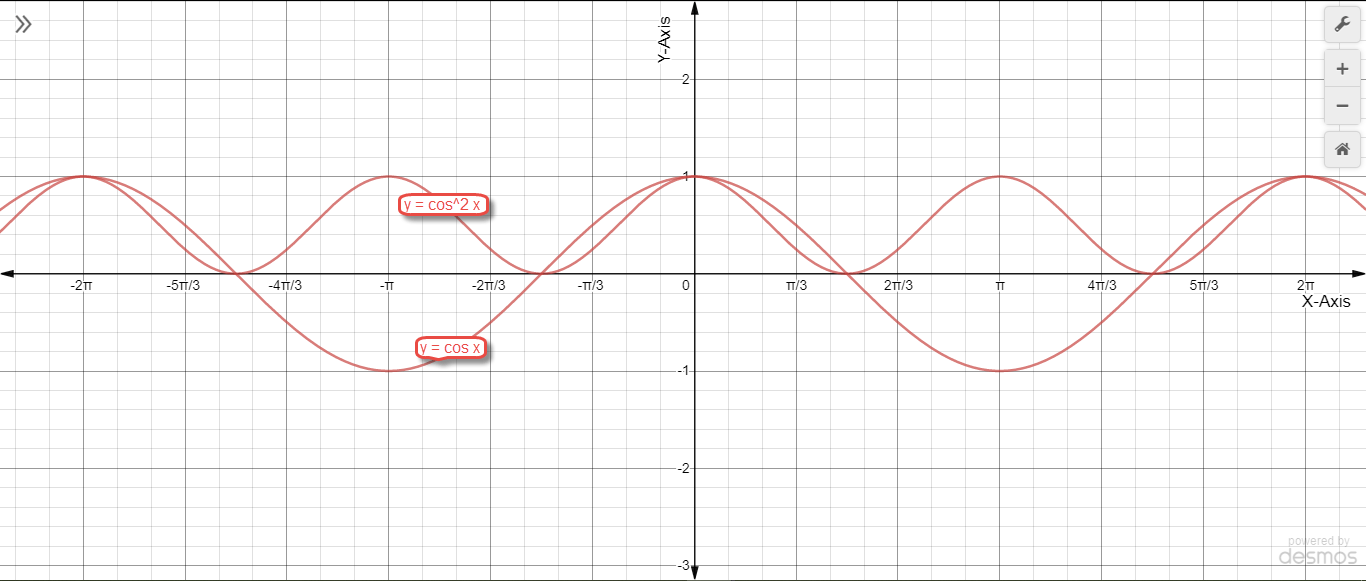Question 1:$\displaystyle \text{i) } f(x) = \cos \Big( x - \frac{\pi}{4} \Big) \hspace{1.0cm} \text{ii) } f(x) = \cos \Big( x + \frac{\pi}{4} \Big) \hspace{1.0cm} \text{iii) } f(x) = \cos^2 x$$\displaystyle \text{iv) }\text{ } f(x) = 2 \cos \Big( x - \frac{\pi}{6} \Big) \hspace{1.0cm} \text{v) } f(x) = \cos 3x \hspace{1.0cm} \text{vi) } f(x) = \cos^2 \frac{x}{2}$$\displaystyle \text{vii) } f(x) = \cos \pi x \hspace{1.0cm} \text{viii) } f(x) = \cos 2\pi x$$\displaystyle \text{i) } f(x) = \cos \Big( x - \frac{\pi}{4} \Big)$$\displaystyle \text{ii) } f(x) = \cos \Big( x + \frac{\pi}{4} \Big)$$\displaystyle \text{iii) } f(x) = \cos^2 x$$\displaystyle \text{iv) } f(x) = 2 \cos \Big( x - \frac{\pi}{6} \Big)$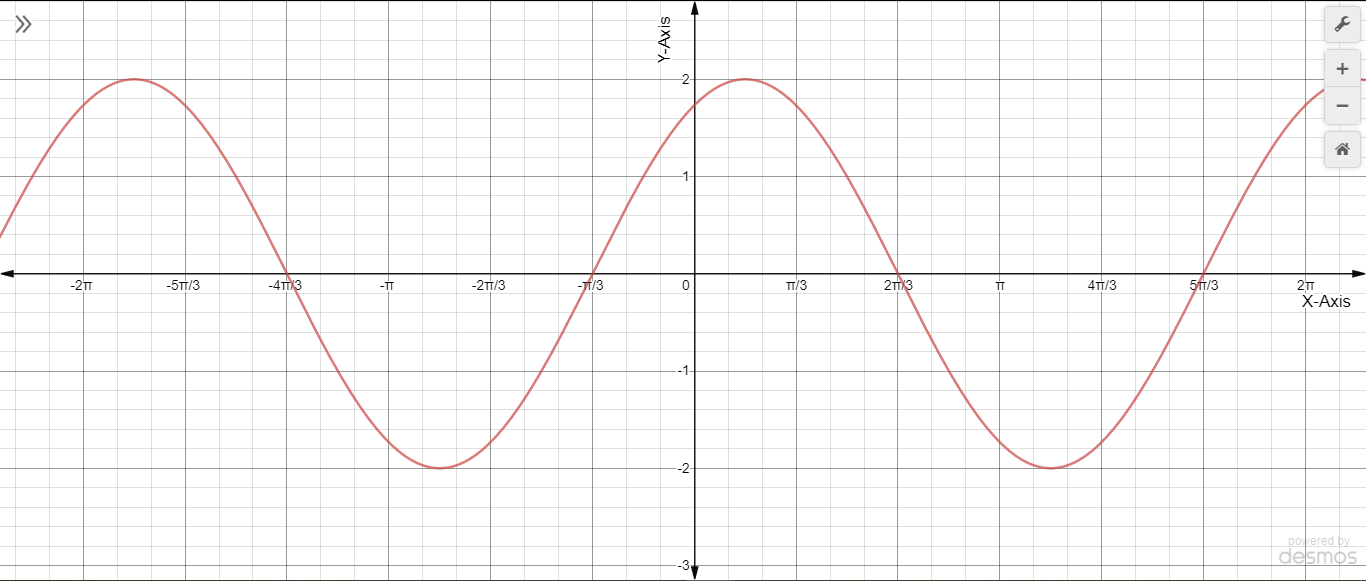$\displaystyle \text{v) } f(x) = \cos 3x$$\displaystyle \text{vi) } f(x) = \cos^2 \frac{x}{2}$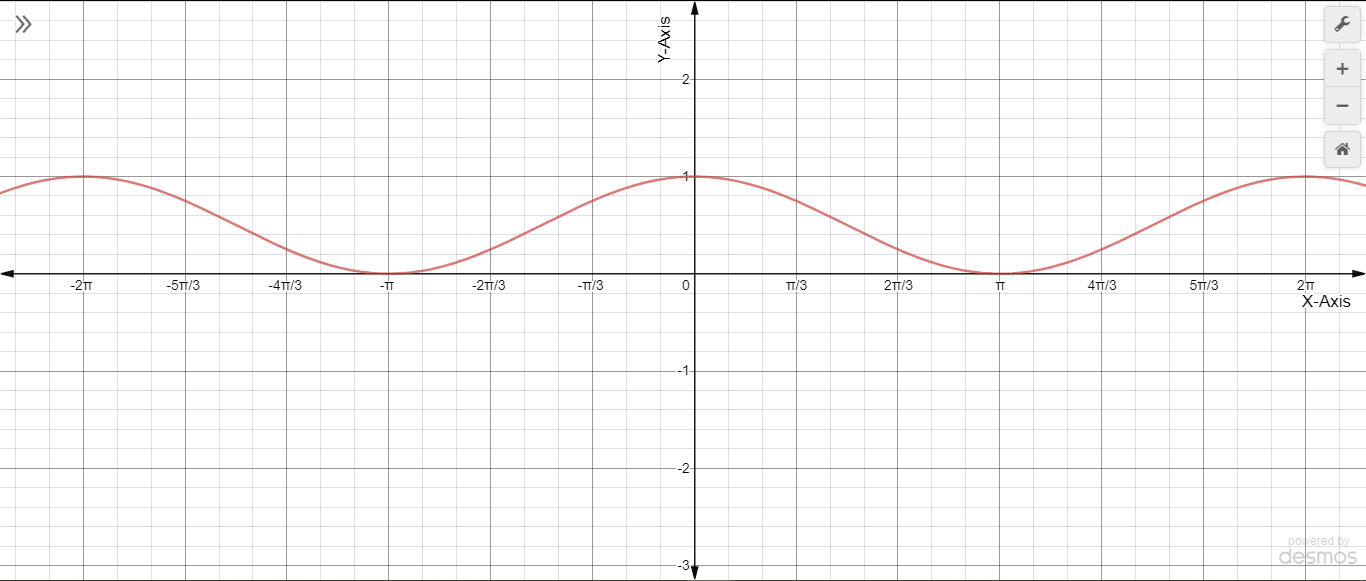$\displaystyle \text{vii) } f(x) = \cos \pi x$$\displaystyle \text{viii) } f(x) = \cos 2\pi x$$\\$

Question 2:$\displaystyle \text{i) } y = \cos x \text{ and } y = \cos \Big( x - \frac{\pi}{4} \Big) \hspace{1.0cm} \text{ ii) } y = \cos 2x \text{ and } y = \cos 2\Big( x - \frac{\pi}{4} \Big)$$\displaystyle \text{iii) } y = \cos x \text{ and } y = \cos \frac{x}{2} \hspace{1.0cm} \text{ iv) } y = \cos^2 x \text{ and } y = \cos x$$\displaystyle \text{i) } y = \cos x \text{ and } y = \cos \Big( x - \frac{\pi}{4} \Big)$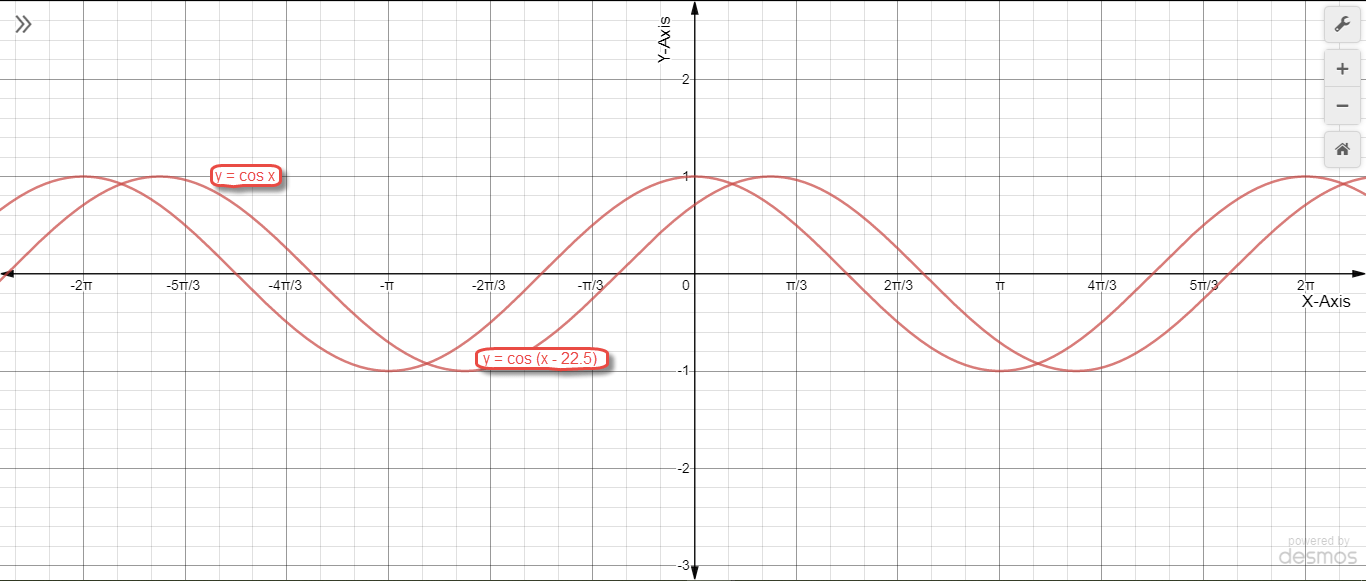$\displaystyle \text{ii) } y = \cos 2x \text{ and } y = \cos 2\Big( x - \frac{\pi}{4} \Big)$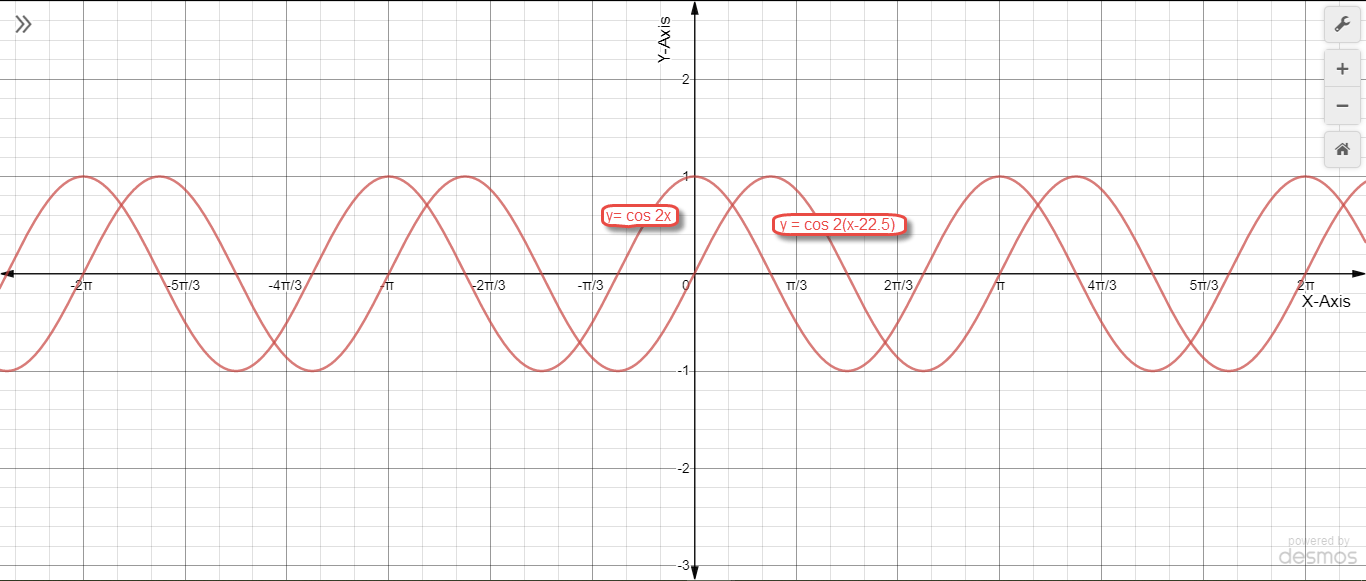$\displaystyle \text{iii) } y = \cos x \text{ and } y = \cos \frac{x}{2}$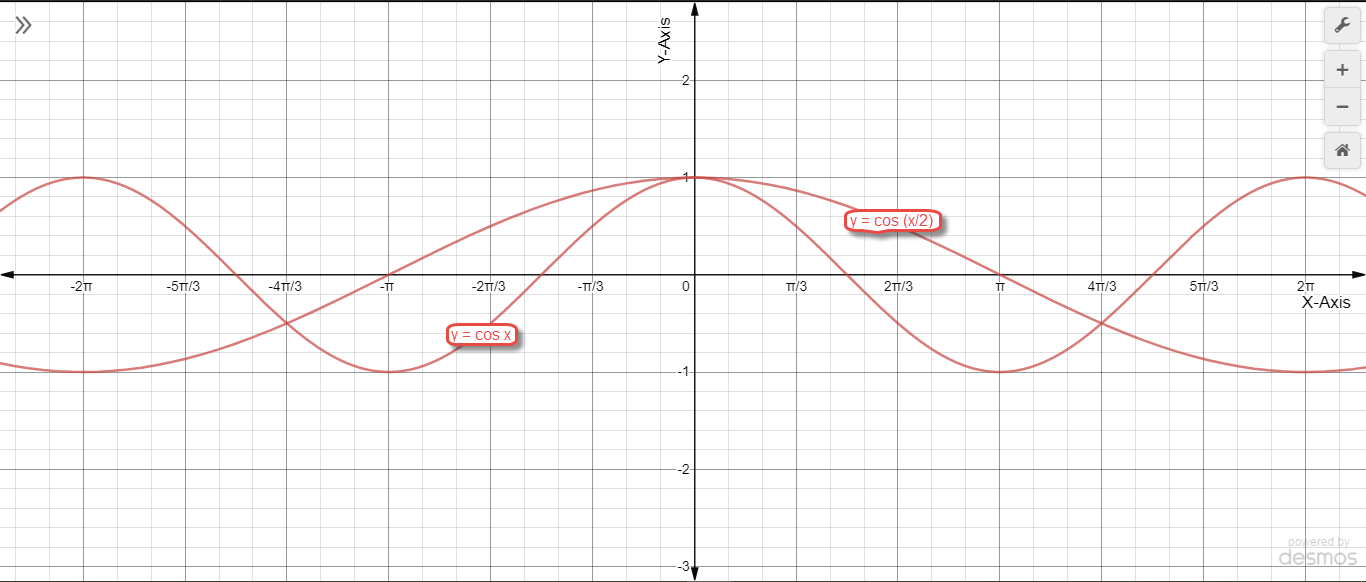$\displaystyle \text{iv) } y = \cos^2 x \text{ and } y = \cos x$